Kinetic Energy and the Law of Conservation of Energy

# Kinetic Energy and the Law of Conservation of Energy - Science Class 9

### Kinetic Energy

A moving object is capable of doing work because of it" motion. Hence, we say that the object has kinetic energy. "Kinetikos" in Greek means "to move". Hence,kinetic energy means energy due to motion. The energy is stored in the object when work is done to change its velocity from a lower value to a higher value, or from rest to certain velocity.

Kinetic energy of an object is defined as the energy which it possesses by virtue of its motion, and is measured by the amount of work that the object can do against an opposing force before it comes to rest.

Kinetic energy of an object moving with a certain velocity is equal to the work done on it to enable it to acquire that velocity.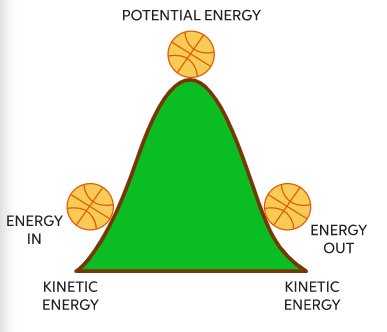### Examples of bodies possessing Kinetic Energy

1. A ball rolling on a surface because it can set another ball into motion by striking it.

2. A bullet fired from a gun as it is able to penetrate some distance into a target which it strikes.

3. A tarpedo in motion as it can do work by penetrating into the side' of a ship.

4. Water in motion as it can turn a wheel or a turbine.

5. A fast wind as it can set a boat in motion when striking against its sail.

6. A moving hammer as it drives a nail into a wall against the resistance offered to it by the wall.

7. A falling body as it can break something on which it falls.

### Expression for Kinetic Energy

Consider an object of mass m which is moving with an initial velocity u on a perfectly frictionless surface. Let a constant external force F act on it and produce an acceleration a in it. If v is the final velocity of the object after having undergone a displacements, then from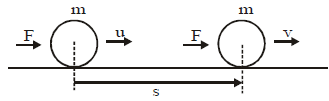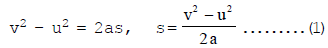Work done by the force in displacing the body through s, i.e.,

W= F × s ..........(2)

We know from Newton's Second Law of Motion,

F = ma ...........(3)

From equation (1), (2) and (3), we get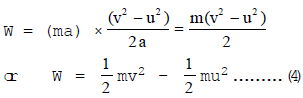If the object is initially at rest, u = 0 and as such from eqn. (4),

W = (1/2)mv2 ...........(5)

This work done (W) in making the object acquire a velocity v after starting from rest has not gone waste and is, in fact, stored in the object.

Work stored up in a moving object is called the kinetic energy of the object.

If kinetic energy of an object is denoted by Ek then

Ek =(1/2})mv2 ..............(6)

Kinetic energy of a moving object is defined as half the product of the mass of the object and the square of the speed of the object.

### Work-Energy Theorem

The work-energy theorem states that the net work done by a moving body can be calculated by finding the change in KE.

⇒ W net = KE final − KE initial

⇒ Wnet= 1/2 x m[v2−u2]

• Mass
• Velocity
• Momentum

## Potential Energy

The energy possessed by an object by virtue of its position or configuration is called its potential energy. It is measured by the work that the object can do in passing from its present position or configuration to some standard position or configuration (known as zero position or zero-configuration).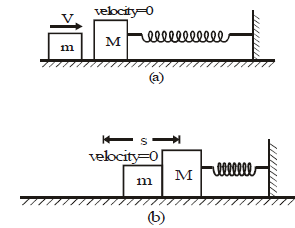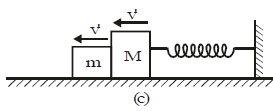The kinetic energy of mass m converts into elastic potential energy of spring.
Example:

Let a small mass m be released from a smooth inclined plane. Another mass M is kept at a rough horizontal plane at rest. The mass m will move along the inclined plane and strike the mass M. Both the masses will move along the horizontal surface for some distance and come to rest. The mass M moves a distance s by the force applied by m. Thus, m does work for which it requires energy. This energy is possessed by m at A as it was at a height h from the horizontal surface. This energy due to position is called potential energy. Precisely speaking this energy is called gravitational potential energy.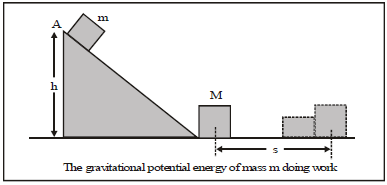Thus potential energy is defined as follows:

The energy possessed by a body due to its position or change in shape is called potential energy.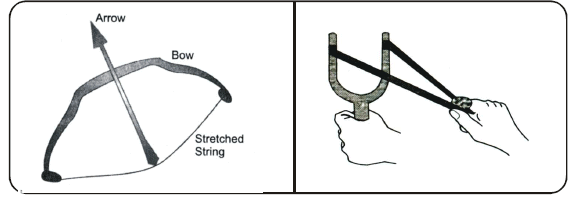Note: The energy possessed by a body due to its height from the surface of earth is called gravitational potential energy and that due to change in shape is called elastic potential energy.

Other examples where elastic potential energy is stored are:
(i) a stretched bow
(ii) a stretched rubber band
(iii) a wound spring
All above examples are because of change in shape.

## Potential Energy of an Object at a Height

Let us consider a block of mass m kept on the surface of earth. Let the block be lifted to a height h. For that a force F is required which is equal to mg.

This force lifts the block through a distance h. The work done by this force,

∴  W = mg × h [ ∵ F = mg]W = F × h

This work is converted into potential energy (P.E.) of the block.

P.E = mgh

The expression shows that potential energy depends on
(a) mass m
(b) height h from ground
(c) acceleration due to gravity g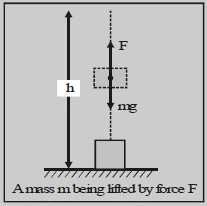## Law of Conservation of Energy

According to law of conservation of energy, energy can neither be created nor destroyed, it can be converted from one form to another.

### Conservation of Mechanical Energy

Let us consider two cases where mechanical energy is conserved.

Case: A ball is dropped from some height.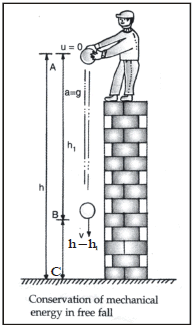At point A: Let a ball of mass m is dropped from a height h. Here the total energy (T.E.) of the ball is the sum of kinetic energy (K.E.) and potential energy (P.E.).

Potential energy = mgh

Kinetic energy = 1/2 m (0)2 = 0 (u = 0)

∴ [T.E.]A = mgh 0 = mgh ... (A)

At point B: Let the ball travel a distance of h1 in time t during its fall. Then the velocity of the ball after time t can be found by using equation of motion. u = 0, a = g, S = h1, v = v

Using v2 - u2 = 2as

v2 - u2 = 2gh1

⇒ v2 = 2gh1

Now K.E. at B =  1/2 mv2  = 1/2m × 2gh1 = mgh1

P.E. at B = mg (h - h1)

Total energy at B = K.E. + P.E. = mgh1  + mg(h - h1) = mgh ... (B)

At point C: Suppose the ball cover a distance h when it moves from A to C. Let V be the velocity of the ball at point C just before it thouches the ground, then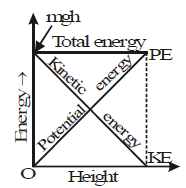v2 - u2 = 2gh

v2 - 0 = 2gh or v2 = 2gh therefore

Kinetic energy (K.E.) = 1/2 mv2 = 1/2 m (2gh) = mgh

and potential energy at C      ∴ P.E. = 0

Hence total energy at point C.

E = K.E. + P.E. = mgh 0 = mgh ... (C)
Thus it is clear from equations A, B and C, that the total mechanical energy of a freely falling ball remain constant. There is, simply, a transformation of mechanical energy. This transformation is depicted in the graph of figure.

The document Kinetic Energy and the Law of Conservation of Energy | Science Class 9 is a part of the Class 9 Course Science Class 9.
All you need of Class 9 at this link: Class 9

## Science Class 9

66 videos|352 docs|97 tests

## FAQs on Kinetic Energy and the Law of Conservation of Energy - Science Class 9

 1. What is potential energy?Ans. Potential energy is the energy possessed by an object due to its position or condition. It is stored energy that has the potential to do work.
 2. How is potential energy related to an object's height?Ans. Potential energy of an object at a height is directly related to the height and the mass of the object. The higher the object is placed, the greater its potential energy.
 3. What is the law of conservation of energy?Ans. The law of conservation of energy states that energy cannot be created or destroyed, but it can be transferred or transformed from one form to another. In the case of potential energy, it can be converted into kinetic energy when the object starts moving.
 4. How is kinetic energy related to the law of conservation of energy?Ans. Kinetic energy is the energy of motion. According to the law of conservation of energy, the total energy of a closed system remains constant. Therefore, the potential energy of an object can be converted into kinetic energy, or vice versa, while the total energy of the system remains constant.
 5. What are some examples of potential energy?Ans. Some examples of potential energy include a stretched elastic band, a raised weight, a compressed spring, or a book placed on a shelf. In each of these cases, the objects possess potential energy due to their position or condition.

## Science Class 9

66 videos|352 docs|97 testsExplore Courses for Class 9 examSignup to see your scores go up within 7 days! Learn & Practice with 1000+ FREE Notes, Videos & Tests.
10M+ students study on EduRev
Track your progress, build streaks, highlight & save important lessons and more!
Related Searches

,

,

,

,

,

,

,

,

,

,

,

,

,

,

,

,

,

,

,

,

,

;